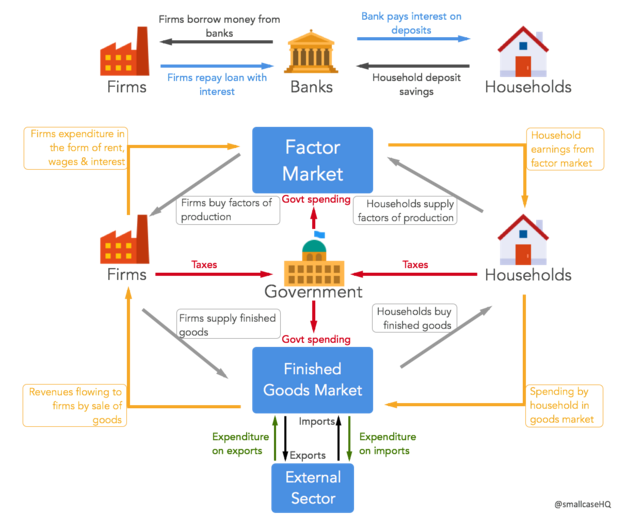# Measuring GDP

Understanding how to measure gross domestic produce (GDP) becomes very easy after learning circular flow of income. We recommend reading our previous three articles on circular flow of products and money before continuing on. As discussed in an earlier article, GDP is the value of final goods and services produced domestically in a given time period. When we say GDP of India was USD 2.3 trillion in financial year 2015, we mean that total value of all goods and services produced in the Indian economy between April 2014 and March 2015 was USD 2.3 trillion.

In order to measure GDP, we need to calculate the expenditure incurred on producing all the goods and services. Obviously, goods and services produced in the economy will get consumed generating income for producers. From our previous article on circular flow of income we know that income is equal to expenditure. So if we can measure the total spending on goods and services in the economy, we will be able to arrive at the total GDP figure. Hence we can either use the total expenditure or total income approach to calculate total GDP.Let’s discuss the expenditure approach. From the above diagram, we can see that there are 4 major spenders in the economy. Households are spending on purchasing goods and services produced by firms, firms are spending on their factor requirements, Government is spending to create public goods and finally foreigners are spending to buy domestic goods.

Total Expenditure = Household expenditure on goods and service (C) + Firm expenditure on capital and other factors (I)+ Government’s expenditure on public goods (G)+ Foreigners net expenditure on exports (X-M)

GDP = C + I + G + (X – M)

Here, C is the consumption by households, I is defined as the investment by firms, G is the government expenditure and X-M is export minus imports. This is how GDP is measured in the expenditure approach.

If any of the above 4 things increase, GDP of the country will also increase. If households are consuming more because of rising levels of income, then GDP will increase. If firms are investing more, because borrowing money has become easy due to low interest rates, then GDP will increase. If Government is spending more on public goods, then GDP will increase. Finally, if exports are rising faster than imports, even then GDP will increase.

The above concept will become clearer in the next posts. Read on.